Courses

# RD Sharma Solutions - Ex-15.4, Properties Of Triangles, Class 7, Math Class 7 Notes | EduRev

## Class 7: RD Sharma Solutions - Ex-15.4, Properties Of Triangles, Class 7, Math Class 7 Notes | EduRev

The document RD Sharma Solutions - Ex-15.4, Properties Of Triangles, Class 7, Math Class 7 Notes | EduRev is a part of the Class 7 Course RD Sharma Solutions for Class 7 Mathematics.
All you need of Class 7 at this link: Class 7

Q1. In each of the following, there are three positive numbers. State if these numbers could possibly be the lengths of the sides of a triangle:

(i) 5, 7, 9

(ii) 2, 10.15

(iii) 3, 4, 5

(iv) 2, 5, 7

(v) 5, 8, 20

Solution.

(i) Yes, these numbers can be the lengths of the sides of a triangle because the sum of any two sides of a triangle is always greater than the third side. Here, 5+7>9, 5+9>7, 9+7>5

(ii) No, these numbers cannot be the lengths of the sides of a triangle because the sum of any two sides of a triangle is always greater than the third side, which is not true in this case.

(iii) Yes, these numbers can be the lengths of the sides of a triangle because the sum of any two sides of triangle is always greater than the third side. Here, 3+4 >5, 3+5> 4, 4+5> 3

(iv) No, these numbers cannot be the lengths of the sides of a triangle because the sum of any two sides of a triangle is always greater than the third side, which is not true in this case. Here, 2 + 5 = 7

(v) No, these numbers cannot be the lengths of the sides of a triangle because the sum of any two sides of a triangle is always greater than the third side, which is not true in this case. Here, 5 + 8 <20

Q2. In Fig, P is the point on the side BC. Complete each of the following statements using symbol ‘ =’,’ > ‘or ‘ < ‘so as to make it true:

(i) AP… AB+ BP

(ii) AP… AC + PC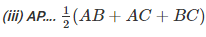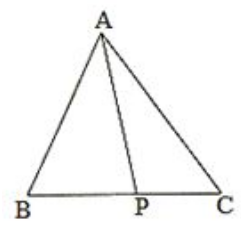Solution.

(i) In triangle APB, AP < AB + BP because the sum of any two sides of a triangle is greater than the third side.

(ii) In triangle APC, AP < AC + PC because the sum of any two sides of a triangle is greater than the third side.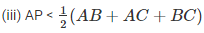In triangles ABP and ACP, we can see that:

AP < AB + BP…(i) (Because the sum of any two sides of a triangle is greater than the third side)

AP < AC + PC…(ii) (Because the sum of any two sides of a triangle is greater than the third side)

On adding (i) and (ii), we have:

AP + AP < AB + BP + AC + PC

2AP < AB + AC + BC (BC = BP + PC)

AP < (AB-FAC+BC)

Q3. P is a point in the interior of ΔABC as shown in Fig. State which of the following statements are true (T) or false (F):

(i) AP+ PB< AB

(ii) AP+ PC> AC

(iii) BP+ PC = BC

Solution.

(i) False

We know that the sum of any two sides of a triangle is greater than the third side: it is not true for the given triangle.

(ii) True

We know that the sum of any two sides of a triangle is greater than the third side: it is true for the given triangle.

(iii) False

We know that the sum of any two sides of a triangle is greater than the third side: it is not true for the given triangle.

Q4. O is a point in the exterior of ΔABC. What symbol ‘>’,’<’ or ‘=’ will you see to complete the statement OA+OB….AB? Write two other similar statements and show that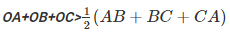Solution.

Because the sum of any two sides of a triangle is always greater than the third side, in triangle OAB, we have:

OA+OB> AB —(i)

OB+OC>BC —-(ii)

OA+OC > CA —–(iii)

On adding equations (i), (ii) and (iii) we get :

OA+OB+OB+OC+OA+OC> AB+BC+CA

2(OA+OB+OC) > AB+BC +CA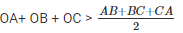Q5. In △ABC, ∠B=30, ∠C=50. Name the smallest and the largest sides of the triangle.

Solution.

Because the smallest side is always opposite to the smallest angle, which in this case is 30, it is AC. Also, because the largest side is always opposite to the largest angle, which in this case is 100,, it is BC.

The document RD Sharma Solutions - Ex-15.4, Properties Of Triangles, Class 7, Math Class 7 Notes | EduRev is a part of the Class 7 Course RD Sharma Solutions for Class 7 Mathematics.
All you need of Class 7 at this link: Class 7Use Code STAYHOME200 and get INR 200 additional OFF Use Coupon Code
All Tests, Videos & Notes of Class 7: Class 7

### Top Courses for Class 7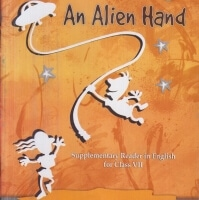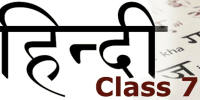## RD Sharma Solutions for Class 7 Mathematics

97 docs

### Top Courses for Class 7Track your progress, build streaks, highlight & save important lessons and more!

,

,

,

,

,

,

,

,

,

,

,

,

,

,

,

,

,

,

,

,

,

,

,

,

,

,

,

,

,

,

;